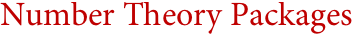Wolfram Archive
Archived 2008. This page no longer reflects the current scope and capabilities of Wolfram's technologies. Find out more about the latest version of Mathematica »

# Number Theory Packages(PARI/GP, Magma, Kant, NTL, ...)
Mathematica not only has broad and deep coverage of state-of-the-art number theory, but also immediately integrates number theory into the complete Mathematica computation and interface environment. Mathematica's unequaled web of symbolic, numeric and other algorithms has made possible a new generation of number theoretic functionality, with many original algorithms developed at Wolfram Research. Mathematica's number theoretic functions are fully scalable, and can immediately be used in high-performance computing, and with gridMathematica.
Number Theory Package Features Built into Mathematica:
Key Advantages of Mathematica for Number Theory:
Interoperability with Number Theory Packages:
Interesting Tidbits:
See Also Analyses On: